Function Repository Resource:

# SolveSudokuPuzzle

Solve a sudoku puzzle

Contributed by: Paritosh Mokhasi
 ResourceFunction["SolveSudokuPuzzle"][mat] solves a sudoku puzzle with the input mat specified by a SparseArray matrix.

## Details and Options

The input matrix must be a SparseArray square matrix with the row length a square integer. All input values must be integers with a magnitude between 1 and the puzzle dimension.
If an element in the matrix is negative, then ResourceFunction["SolveSudokuPuzzle"] solves the puzzle assuming that the specified number cannot exist at that position.
The conditions that define a solution for dimension dim are that all integers in a row or column be distinct (a permutation of 1dim) and that subsquares of size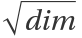also be a permutation of that set.
If the input has no solution, then it is returned unevaluated.
If there are multiple solutions, ResourceFunction["SolveSudokuPuzzle"] returns one of them.

## Examples

### Basic Examples (2)

Solve a standard Sudoku puzzle:

 In:=Out=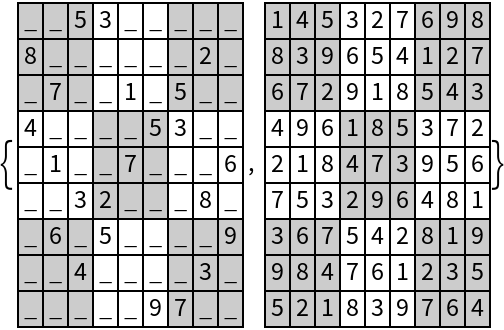Solve a 1616 Sudoku puzzle:

 In:=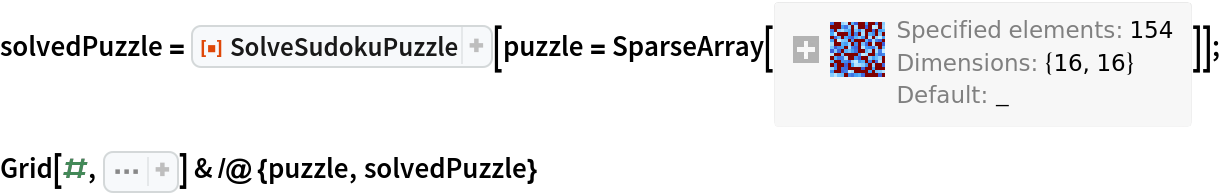Out=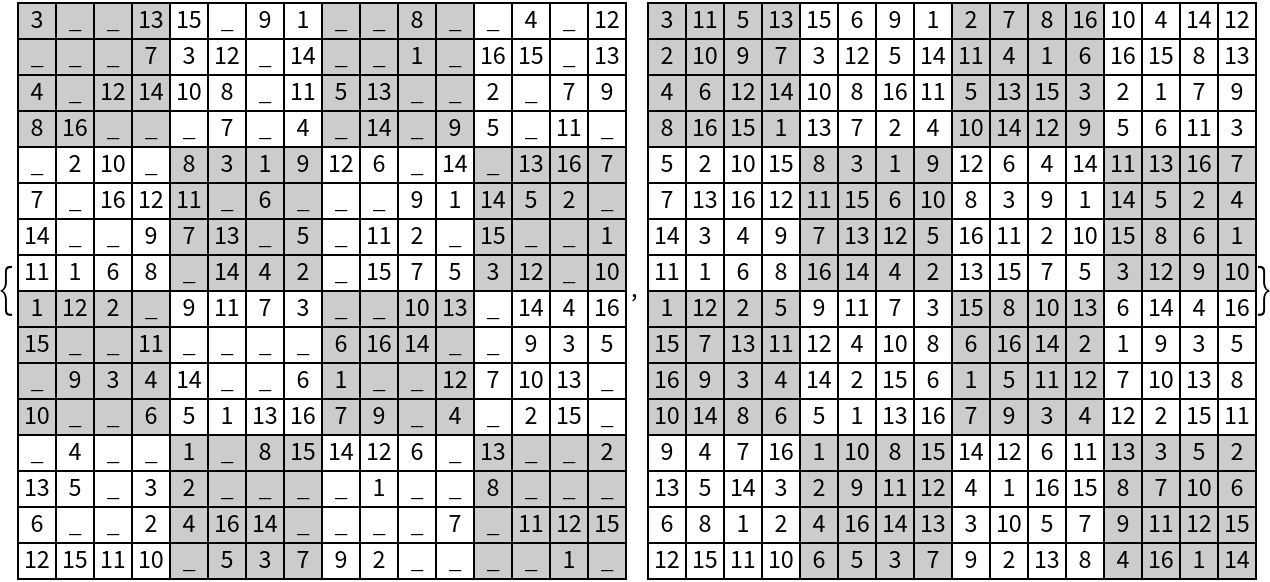### Scope (3)

Generate a full standard Sudoku board by specifying the diagonal elements:

 In:=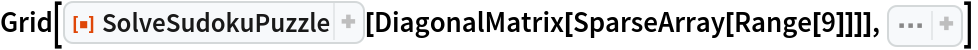Out=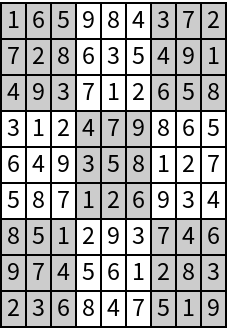Generate a full 1616 Sudoku board by randomly specifying the first column:

 In:=Out=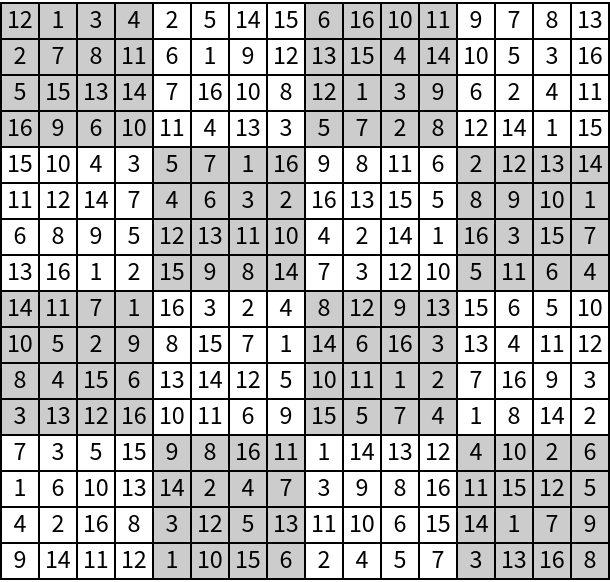Generate a Sudoku board by specifying elements that cannot exist at certain positions:

 In:=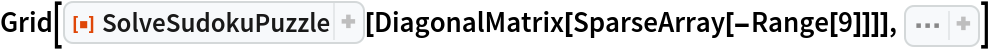Out=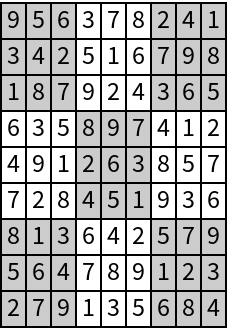Paritosh Mokhasi

## Version History

• 1.0.0 – 18 May 2020